# 抓取京东JSRC安全技术文章自动化生成word文档

### 抓取京东JSRC安全技术文章自动化生成word文档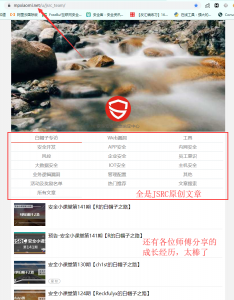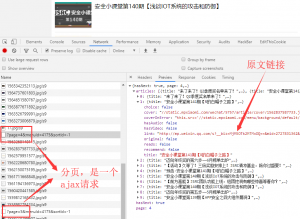```def get_xvlink():
for i in range(1,23):
print('正在抓取第{}页的链接'.format(str(i)))
for i in info:
f.close()```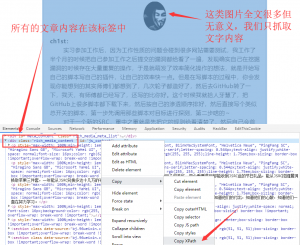```def get_content(url):
text = ''
p = r.html.xpath('//*[@id="js_content"]',first=True).text.split('\n')
p = [i for i in p if i !='']
for i in p:
if len(i) &lt; 20:
text += i
else:
text += i+'\n'
return text```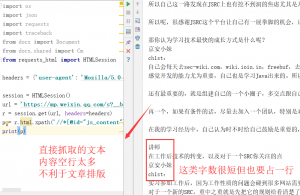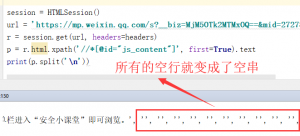```def generate_word(title,text):
document = Document()    #定义一个文档对象
sections = document.sections
for section in sections:   #调整页边距
section.top_margin = Cm(0)
section.bottom_margin = Cm(0)
section.left_margin = Cm(1)
section.right_margin = Cm(1)
document.save(title+'.docx')
print('文章'+title+'word文档生成完毕！')```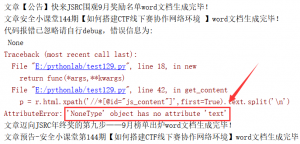```import os
import json
import requests
import traceback
from docx import Document
from docx.shared import Cm
from requests_html import HTMLSession

headers = {'user-agent': 'Mozilla/5.0 (Windows NT 10.0; Win64; x64) AppleWebKit/537.36 (KHTML, like Gecko) Chrome/77.0.3865.120 Safari/537.36'}

session = HTMLSession()

def get_exception(func):
def new(*args,**kwargs):
try:
return func(*args,**kwargs)
except Exception as e:
print('代码报错已忽略请自行debug，错误信息为:\n',traceback.print_exc())
return new

@get_exception
def generate_word(title,text):
document = Document()
sections = document.sections
for section in sections:
section.top_margin = Cm(0)
section.bottom_margin = Cm(0)
section.left_margin = Cm(1)
section.right_margin = Cm(1)
document.save(title+'.docx')
print('文章'+title+'word文档生成完毕！')

@get_exception
def get_content(url):
text = ''
p = r.html.xpath('//*[@id="js_content"]',first=True).text.split('\n')
p = [i for i in p if i !='']
for i in p:
if len(i) &lt; 20:
text += i
else:
text += i+'\n'
return text

@get_exception
for i in range(1,23):
print('正在抓取第{}页的链接'.format(str(i)))
for i in info:
f.close()

def check_path():
path = './JSRC文章合辑'
if not os.path.exists(path): os.mkdir(path)
os.chdir(path)

def main():
for url in urls:
(title,url) = url.split('&amp;&amp;&amp;&amp;&amp;')
text = get_content(url)
generate_word(title,text)

if __name__ == '__main__':
check_path()
main()```JSRC文章合辑.zip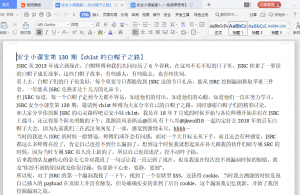微信赞赏支付宝赞赏

mark

哈哈